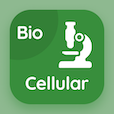SAT Online Courses

SAT Chemistry MCQs

SAT Chemistry MCQ PDF - Topics

# Molarity MCQ Quiz Online

Learn Molarity Multiple Choice Questions (MCQ), Molarity quiz answers PDF to study sat chemistry online course for sat chemistry classes. Solutions Multiple Choice Questions and Answers (MCQs), Molarity quiz questions for questions to ask during an interview. "Molarity MCQ" PDF Book: solutions test prep for SAT subject test.

"To calculate the volume of solvent, we need to know the" MCQ PDF: molarity with choices density of solute, normality of solute, mass of solute, and molarity for questions to ask during an interview. Study molarity quiz questions for merit scholarship test and certificate programs for free career quiz.

## MCQs on Molarity Quiz

MCQ: To calculate the volume of solvent, we need to know the

Density of solute
Normality of solute
Mass of solute
Molarity

MCQ: Molarity of pure water is

33.3
55.5
44.4
6.1

MCQ: The number of moles of solute dissolved per dm3 of the solution is

Molality
Molarity
Normality
None of above

MCQ: The molarity of 2% w/v NaOH solution is

0.25
0.5
0.1
0.05

### More Topics from SAT Chemistry Course